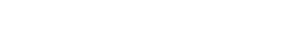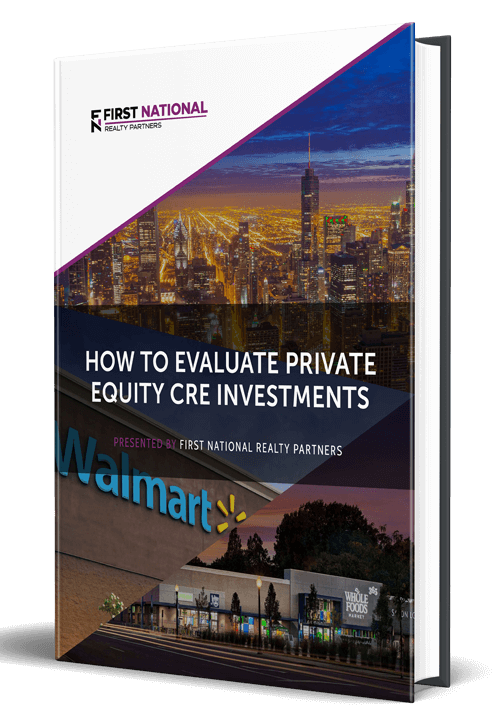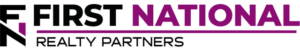# Understanding the Three Ways that Commercial Real Estate (CRE) Lenders Calculate Interest Due

### Key Takeaways

• Nearly all commercial real estate transactions involve debt financing. The terms of the debt can have a material impact on the amount of money that is available to distribute to investors. For this reason, it is important that investors understand every detail of the transaction, particularly the interest accrual method.
• There are three commonly used interest accrual methods: 30/360, Actual / 360, and Actual 365.
• The differences between the three have to do with how many days of interest accrue each month. The result determines how much interest is due with each monthly payment.

One of the major benefits of commercial real estate investment is the wide availability of commercial mortgage loans at relatively favorable terms. As a result, individual real estate investors can minimize the amount of money that they must invest in a transaction, which boosts the return on the cash they receive.

However, every commercial real estate loan transaction is different. There are many different types of loans, and the terms can vary based on many different factors—including the creditworthiness of the borrower and the financial statements for the collateral property.

It is absolutely critical that investors understand every detail of the repayment arrangement. One of the trickier aspects of a commercial loan is how the lender calculates the amount of interest due. There are three methods that are commonly used by financial institutions and the differences can have a significant impact on the amount of interest paid over the life of the loan. Consequently, it can also have an impact on the amount of cash flow that is available to be distributed to property investors.

To illustrate how the different methods work, it is helpful to set up a scenario.

## Loan Scenario

Suppose that an investor is considering the purchase of a commercial property for \$1,000,000. As a potential borrower, they have qualified for a loan with the following terms at origination:

• Loan Amount: \$750,000 (75% Loan to Value or LTV)
• Down Payment: \$250,000
• Loan Term: 25 years
• Amortization Period: 25 years
• Interest Rate: 5.00%
• Number of Payments per Year: 12 (Monthly Payments)

The payments will consist of principal and interest monthly. To calculate the interest component, there are three methods that the lender could use: 30/360, Actual/365, and Actual/360. The differences between each are the periods of time over which interest accrues, and these differences are discussed in detail below.

## 30/360 Days

With the 30/360 interest accrual method, it is assumed that there are 30 days in every month and 360 days in the year. Based on the parameters described above, the mortgage payment is \$5,845 per month. The interest component for the first payment can be calculated in three steps.

First, the annual interest rate is divided by 360 to get the daily rate. 5% divided by 360 days equals .013889%.

Second, the daily accrual rate is multiplied by 30 days in the month to get .041667%. This is the monthly accrual rate.

Finally, the monthly accrual rate is multiplied by the loan balance of \$1,000,000. The result is \$4,166. This is the interest component of the first loan payment.

To calculate the principal components, the interest component is subtracted from the payment to get \$1,679 (\$5845 – \$4,166).

For the purpose of this article, the key number in this calculation is the \$4,166 in interest paid in the first month. This will be compared to the other options.

## Actual/365 Days

With the Actual / 365 days methodology, interest is accrued based on the actual number of days in the month and the assumption that there are 365 days in the year. The calculation follows the same three-step pattern as above.

First, the annual interest rate is divided by 365 days to get the daily accrual rate. The 5% divided by 365 days equals .013699%—slightly less than in the first scenario.

Second, the daily accrual rate is multiplied by the actual number of days in the month. For this example, assume that the first payment occurs in January, which has 31 days. So, .013699 * 31 is equal to .0424658%.

Finally, this number is calculated by the outstanding balance of \$1,000,000 to get the monthly interest payment of \$4,246 for the first month’s payment.

The key figure from this calculation is \$4,246. Immediately, it can be seen that there is a difference of \$80.57 between the 30/360 and actual/365 accrual methods.

## Actual/360 Days

The pattern in calculation should be clear by now. With this methodology, interest is accrued based on the actual number of days in the month and the assumption of 360 days in a year. The calculation follows the same three steps.

First, the annual interest rate of 5% is divided by 360 to get the daily accrual rate. The 5% divided by 360 equals .013889%.

Second, this rate is multiplied by the actual number of days in the month. Again, assume that the first payment is made in January, which has 31 days. .013889 * 31 is equal to .0430556%. This is the monthly accrual rate.

Finally, multiply the monthly accrual rate by the outstanding loan balance of \$1,000,000 and the result is \$4,305, which is the key figure from the calculation using this accrual methodology.

## Comparing The Results

Using the scenario above, the monthly interest accrual calculation can be compared using all three calculation methods:

• 30/360: \$4,166
• Actual/365: \$4,246
• Actual/360: \$4,305

From this comparison, it can be seen that there are material differences in the monthly results. While \$80 or \$90 may not seem significant, when this amount is spread over a 25 year loan term, the difference is magnified. The following bullet points describe the total interest paid for the term of the loan using each of the three accrual methods (this can be calculated from a complete amortization schedule):

• 30/360: \$440,753
• Actual/365: \$440,964
• Actual/360: \$449,017

The difference between the lowest and highest amounts is nearly \$9,000, which represents money that is paid to the lender, not to investors. As a result, there are several financial metrics that can be affected including the debt service coverage ratio (DSCR) and overall returns.

So, the key point from this article is that investors should understand which interest accrual method is used in their loan and adjust their financial models accordingly. In most cases, it won’t be possible to negotiate the preferred option, but it is good information to know while underwriting the investment.

## Interested In Learning More?

First National Realty Partners is one of the country’s leading private equity commercial real estate investment firms. We leverage our decades of expertise and our available liquidity to find world-class, multi-tenanted assets below intrinsic value. In doing so, we seek to create superior long-term, risk-adjusted returns for our investors while creating strong economic assets for the communities we invest in.

Get instant access to all of our current and past commercial real estate deals.## Subscribe Now

Get the latest news on real estate

Operations and Management

### October 9, 2023

FNRP Spotlight Series

1031 Exchange

## Get More From FNRP## How to Evaluate Private Equity CRE Investments## How to Complete a 1031 Exchange with a Private Equity Sponsor